# Analytics Calculations: Table Functions

Moderator, Lightspeed Staff Posts: 81 moderator
edited November 2018
*Note, from this part of the Discussions, we will assume that you're comfortable with starting Calculations. Changing dimensions and measures, changing filters, and some of the basic starter calculations: addition, subtraction, division, multiplication, greater than, less than, equal to, single-criteria IF functions, and multiple criteria IF functions.

If you're in the early stages of your Analytics mastery, you may want to take a quick look at the related Discussions:

Multiplying by percentages in Analytics

Analytics provides lots of functions that perform different mathematical, logical, and table-based calculations. You can find a more in-depth catalogue of available functions on the Looker list of functions and operators.

To make it easier, we could categorize these in five different ways: Cell functionsDate functions, Logic FunctionsMath functions, and Table functions.

In this article, we'll be looking at six of the Table-based functions:
Count, Count Distinct, Index, Match, Offset, Offset List

# Count

Returns a count of all non-null values from a range. Requires just one argument, the range being searched/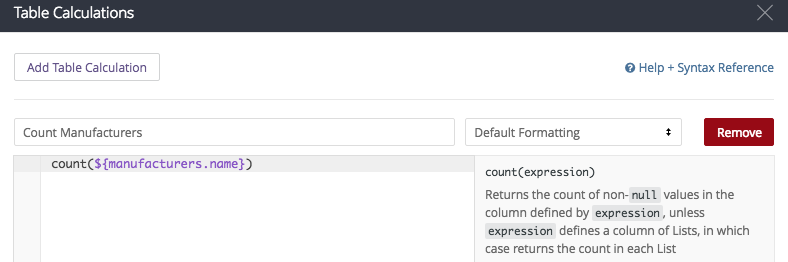So, in this instance, my calculation looks like

count(\${manufacturers.name})

and if I have prepared the calculation properly...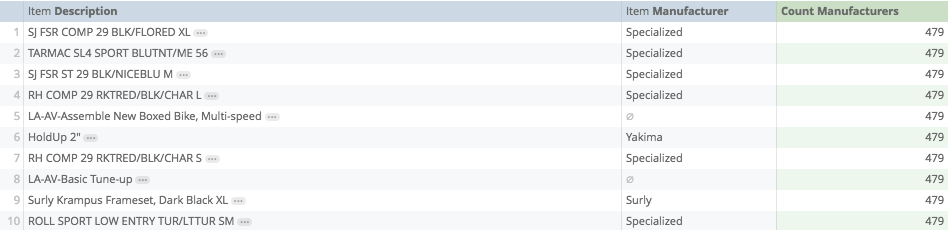# Count Distinct

Counts all unique non-null values from a range, requires one argument, the range being counted.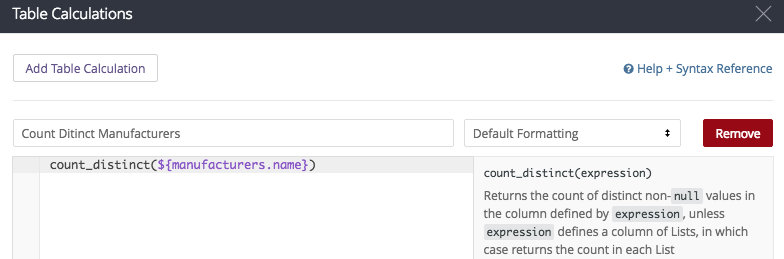In this instance my calculation looks like

count_distinct(\${manufacturers.name})

And if I have prepared my calculation properly, when I save...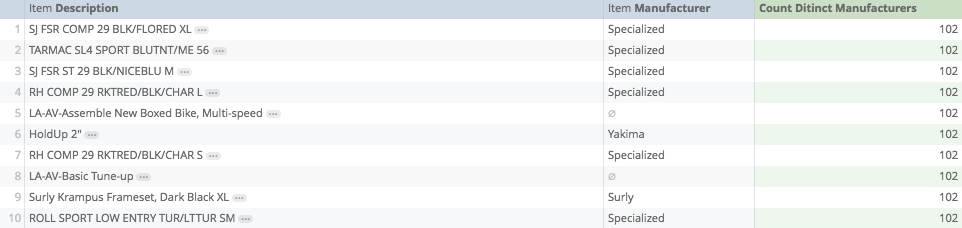# Index

One of the most powerful calculations, returns the content from a specific cell from a defined range. Requires two arguments, the range being looked at, and which cell is being returned:

For example, I could ask the calculation to return the third row from the dates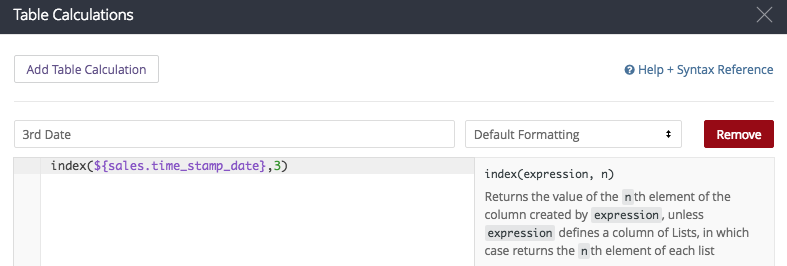in this example, my calculation looks like:

index(\${sales.time_stamp_date},3)

and if I have prepared my calculation properly, when I save: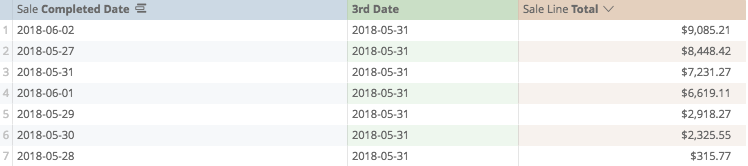# Match

Another powerful tool with Index: returns the row where content is matched. Requires two arguments, which column is being searched, and what content we are looking for.

Say that we are using one calculation to return the largest sale value from the past two weeks, using each row to define the next largest value (sorting from greatest to smallest)

large(\${sale_lines.total_sales_no_tax},row())

Then what we could do, is use a match calculation to find which row that largest value was found on: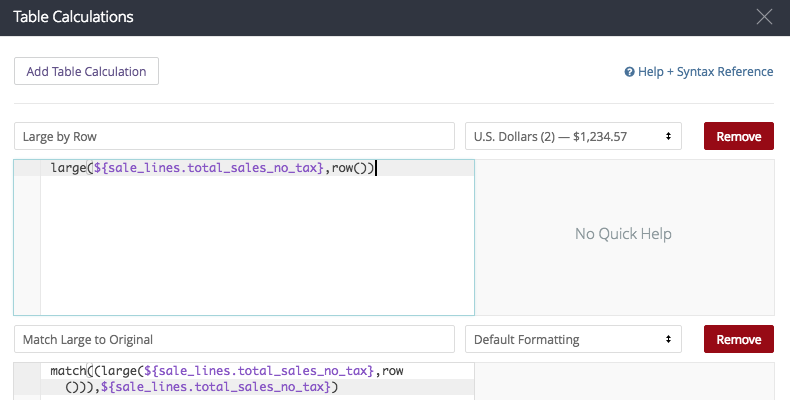so my calculation looks like:

match((large(\${sale_lines.total_sales_no_tax},row())),\${sale_lines.total_sales_no_tax})

and if I have prepared it properly, when I save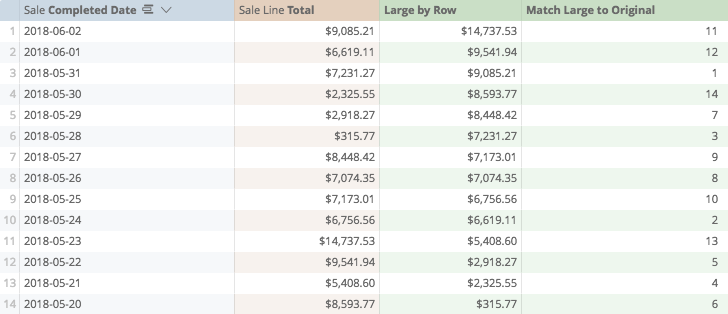While unremarkable on its own, using Match and Index together in compound calculations provides many custom sorting or gathering solutions.

# Offset

Returns the value from a range looking up or down a certain number of rows. Requires two arguments, which column is being looked at, and how many rows down you wish to look

In this instance, I'm using Offset positively to look at the row below, and negatively, to look at the row above...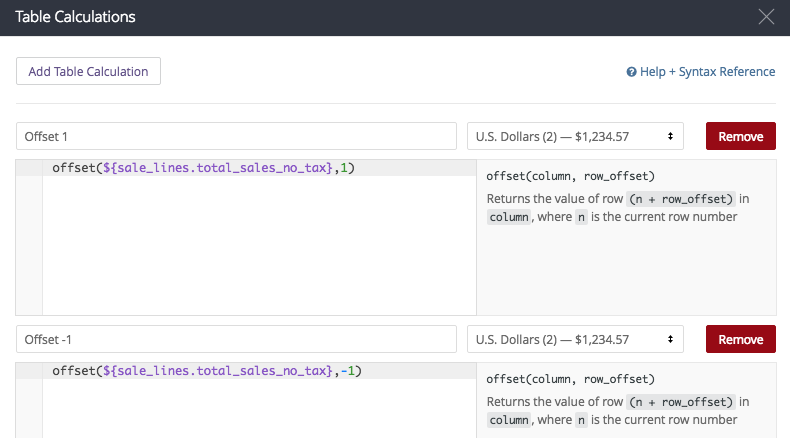my calculations are respectively

offset(\${sale_lines.total_sales_no_tax},1)
offset(\${sale_lines.total_sales_no_tax},-1)

and if I have prepared them properly, when I save...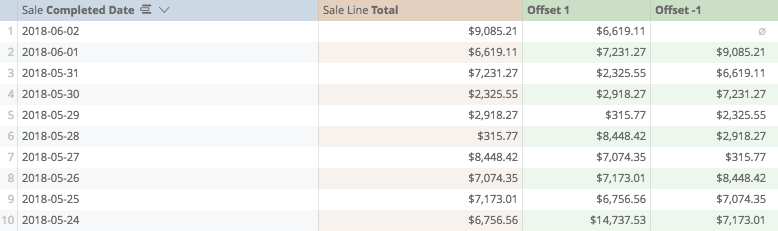# Offset List

Returns a series of numeric or text values from a defined range. Often used to prepare for summing, such as to illustrate a running weekly total of sales.

Requires three arguments: which range to look at, how many rows down you wish to start at, and how many rows you want to include in the list.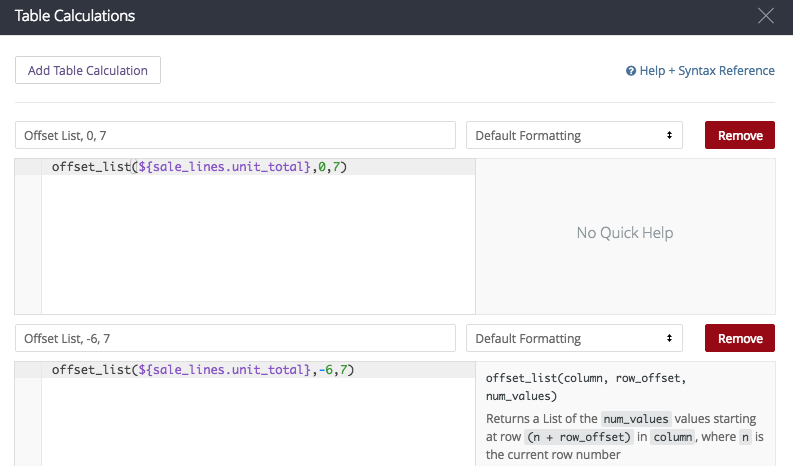In this instance my calculations look like:

offset_list(\${sale_lines.unit_total},0,7)

Which will create a list from this row up to the seventh row down, and

offset_list(\${sale_lines.unit_total},-6,7)

Which will create a list starting six rows back, and ending on the current row

If I have prepared my calculation properly, when I save...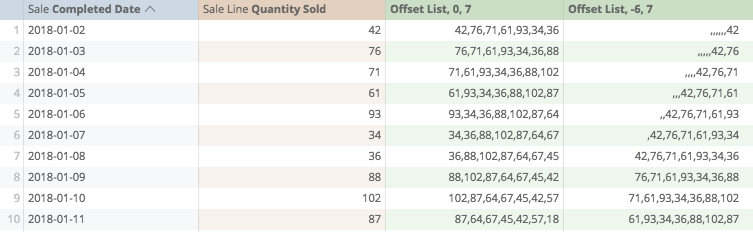Calculations Home
Post edited by josephmckeown on
Joseph McKeown
Lightspeed Analytics and reporting consultant
Lightspeed HQ